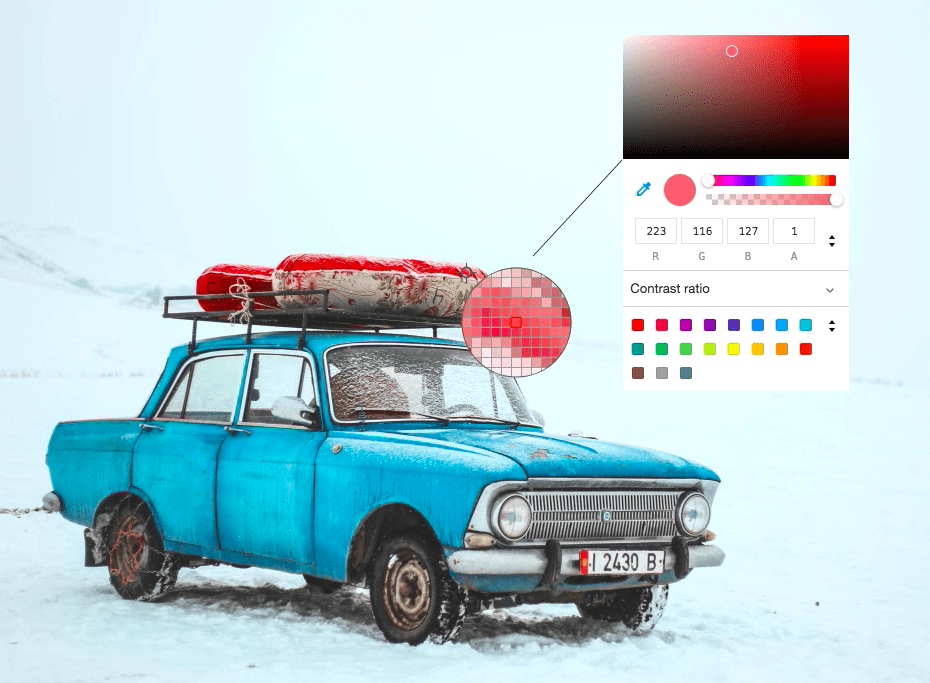# Converting Color Images to Grayscale using numpy and some Mathematics

An extremely magnified image at the end is just blocks of colors called pixels, where each pixel is formed by the combination of Red, Blue and Green, our primary colors. RGB color space or RGB color system, constructs all the colors from the combination of the intensities of Red, Green and Blue colors.The red, green and blue use 8 bits each, which have integer values from 0 to 255. This makes 256*256*256=16777216 possible colors. Below is a chart of basic colors.

Color HTML / CSS Name Hex Code

#RRGGBB

Decimal Code

(R,G,B)

Black #000000 (0,0,0)
White #FFFFFF (255,255,255)
Red #FF0000 (255,0,0)
Lime #00FF00 (0,255,0)
Blue #0000FF (0,0,255)
Yellow #FFFF00 (255,255,0)
Cyan / Aqua #00FFFF (0,255,255)
Magenta / Fuchsia #FF00FF (255,0,255)
Silver #C0C0C0 (192,192,192)
Gray #808080 (128,128,128)
Maroon #800000 (128,0,0)
Olive #808000 (128,128,0)
Green #008000 (0,128,0)
Purple #800080 (128,0,128)
Teal #008080 (0,128,128)
Navy #000080 (0,0,128)

If you look at Gray and Silver, you would see that all the components Red, Green and Blue are equal. So the idea behind converting any image to grayscale would be to make Red, Green and Blue value equal in each pixel. Some of the ways you can do this are given below:

### Method 1: The lightness method

This method averages the most prominent and least prominent Colors: (max(R, G, B) + min(R, G, B)) / 2

`gray_light[:] = np.max(gray_light,axis=-1,keepdims=1)/2 + np.min(gray_light,axis=-1,keepdims=1)/2`

output image:### Method 2: The average method

This method simply averages the values: (R + G + B) / 3

`gray_avg[:] = np.sum(gray_avg,axis=-1,keepdims=1)/3`

output image:### Method 3: The luminosity method

This is a more sophisticated version of the average method. It also averages the values, but it forms a weighted average to account for human perception. We’re more sensitive to green than other colors, so green is weighted most heavily. The formula for luminosity is 0.21 R + 0.72 G + 0.07 B

```luminosity_constant = [0.21,0.72,0.07]
gray_lumin = np.dot(orig_img[...,:3], luminosity_constant).astype(np.uint8)```

output image:You can see the entire source code here: Various ways of converting an image to grayscale

### Method 4: Simply use some API 😉

You can use the standard skimage method to perform the same grayscale conversion.

```from skimage.color import rgb2gray
img_gray = rgb2gray(orig_img)```

The algorithm used within rgb2gray is the luminosity method.Subscribe
Notify of